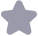### Java经典程序题100例.pdf收藏

Java经典程序题100例，对基础知识数组，集合，各种实例题，来提高大家的基础知识，锻炼编程思维
System. out. printIng for (intj=1:j<10; jl+ or(intk=1;k<10k++){∥老师的做法,判断语句里的k<j,省去下列的讧语句。 if (k>j brcak; ∥|处用 continuc也可以,只是效率低一点 System. out. print("++X++=+j*k); System. out printIn(; 6、输岀所有的水仙花数,把谓水仙化数是指一个数3位数,其各各位数字立方和等于其本 身 例如:153=1*1*1+3*3*3+5*5*5 class DafodilNumber public static void main(String[ args)i System. out. printIn("以下是所有的水仙花数"); int number= 100 ∥由于水仙花数是三位数,故由100开始算起 Int 1,1,k;∥ijk分别为 number的百位、十位、个位 for(int sum; number<1000; number++i i=number/100: j=(number-1*100)/10: k=number-1*100-j*10: sumi+j于j+k*k*k; if(sum==number) System. out printIn(number+is a dafodil number!"); 7、求aaaa+.aaa=? 其中a为1至9之中的个数,项数也要可以指定 import java. util. Scanner; class multinomial i public static void main(String[] args) ∥定义输入的a int how many;∥定义最后的一项有多少个数字 Scanner scanner new Scanner(System. in); System. out. printIn("请输入一个1~9的a值") a= scanner. nextInt(; System. out. printIn("请问要相加多少项?"); how Many=scanner. nextInt(; int sum=0 int al-a;∥用来保存a的初始值 for (int i=1; i<=howMany: i++)i sum=a a=10a+a;∥这表示a的下一项 每次a的下一项都等于前一项*10,再加上刚输入时的a;注意,这时的 经变化了 Systcm out printIn("sum=+sum); 8、求2/1+3/2+53+85+13/8.…前20项之和? class su public static void main(sling args)i doublc sum=0 double fenZ-20, fenhu=1.0;∥初始的分了( (fenZi)=2,分丹( fenhu)=1 for(int i=1; i<=20: 1++i sum + fenzi/ fen mu TenMu= lenZi ∥一项的分母=上一项的分子 fenzi +- fen mu /下一项的分子=上一项的分子加分母 System. out printIn("sum="sum) 9、利用程序输出如下图形 * 串*非串串* class asterisk public static void main(String[] args)i for (int 1=l; 1<=13; 11=2)3 for(int j=l; j<=i&&i+j<=14; j++System. out. print("; 3 Systcm. out. printInG;∥换行 1、计算圆周率 PI=4-4/3+45-4/7 打印出第一个大于3.1415小于3.1416的值 class Pi i public static void main(String[] args)i doublc pi=0;∥定义初始值 double fen zi=4;∥分了为4 double fen mu=1;/第一个4,可看作分母为1的分式,以后的分母每次递增2 for(inti=0;i<100000004+){〃运行老久,减少循环次数会快很多,只是精确 度小些 pi+=(finZi/fcn Mu) enzi*=-1.0;∥每项分了的变化是+4,-4,+4,-4 fen mu+=20;∥分母的变化是1,3,5,7, 每项递加2 System. out. println(pi) 输出结果为pi=3.1415926525880504,应该不精确 12、输入一个数据n,计算斐波那契数列( Fibonacci)的第n个值 112358132134 规律:一个数等于前两个数之和 ∥计算斐波那契数列( Fibonacci)的第n个值 public class Fibonacci& public static void main(String argsi int n= Integer. parselnt(argsOD n1=1;第一个数 intn2=1;/第个数 Int sum=0;/和 if(n<0){ System. out printin("参数错误!"); return f(n<=2) cIsc for(int i-3; i<-n;i++) sum=nl+n2 nl=n2 System. out. println(sum) ∥计算斐波那契数列( Fibonacci)的第n个值 ∥)把整个数列打印出来 public class FibonacciPrint( ublic static void main(String args Di int n=IntegerparseInt(argsOD FibonacciPrint t=new FibonacciPrinto for( int i=1 +){ t print(i); public void prini(int n)i intn1-1;第一个数 intn2=1;第二个数 nt sum=0:/和 if(n<=0){ System. out. println("参数错误!); return, if(n<2){ sum for(int i=3; i<-n; i++)i sum=nl tn2 1=n2 um System. out println(sum 13、求1-1/3+15-1/7+19.的值 求出前50项和值 b,求出最后·项绝对值小于le-5的和值。 15、在屏幕上打印出n行的金字塔图案,如,若n-5,则图案如下: * 冰冰本本木 半米***** 打印金字塔图案 public class Printstar( public static void main(String args) int col-Integer. parseInt(args[O; for(inti=1;i<=col;i++){/i表示行数 /打印空格 (int k=0; k<col-i; k++i System. out. print("") /打印星星 for(int m=0; m2*i-1: m++i System. out. print(2); ut printIng 16、歌德巴赫猜想,任何一个大于六的偶数可以拆分成两个质数的和 打印出所有的可能 ∥仟何一个人于六的偶数可以拆分成两个质数的和 打印出所有的可能 public class Gedebahe& public static void main(String argsi int num-Integer. parselnt(argsoD) if(um<=6){ System. out. printIn("参数错误!"); retur f(num%2!=0){ Systen out println("”参数错误!"); return; Gedebahe g= new Gedebaheo l不是质数,2是偶数,因此从3开始循环 for(int i=3; i< -num/2; i++o if69%20){/如果为偶数,退出本次循环 continue, ∥当i与num-都为质数时,满足条件,打印 r(g is Prime(i)&&g is Prime(nurn-i)) System. out. printIn(+++(num-1+="+num) 第4章数组 1.定义一个int型的一维数组,包含10个元素,分别赋一些随机整数,然后求出所有元素 的最大值, 最小值,平均佰,和佰,并输出出来。 class Array Numberi public static void main(String[] args)i int[ array Number; arrayNumber=new int System. out. printIn("以下是随机的10个整数:") ∥填入随机的10个整数 for (int 1=0; i<array Number length; 1++3 arrayNumberi]=(int)(100*Math.random) System. out. print(array Number[]-"; System. out.printIn(; int max=array Number[O] int min= array Numbcr[0; for (int i=0; i< Number length; i++ if(max arrayNumber[i]) max= arrayNumber[i;〃求最大值 if(min array[iD min= arrayNumber[i];∥求最小值 sum + array Number[i] System.out. println("It F Max="+max+", Min="+min+", Sum="+sum+",Avg="+sum/10.) 2定义个nt型的维数组,包含10个元素,分别赋值为1~10,然后将数组中的元素都 向前移一个位置, 即,a0}=alall=a[2l,最后一个元素的值是原米第一个元素的值,然后输出这个数组。 3.定义一个int型的一维数组,包含40个元素,用来存储每个学员的成绩,循环产生40个 0~100之间的随机整数, 将它们存储到一维数组中,然后统计成绩低于平均分的学员的人数,并输出出来。 4!.(选做)承上题,将这40个成绩按照从高到低的顺序输出出来 5,(选做〕编写程序,将个数组中的元素倒排过来。例如原数组为1,2,3,4,5;则倒 排后数组中的值 为5,4,3,2,1。 6,要求定义一个it型数组a,包含100个元素,保存100个随机的4位数。再定义一个 int型数组b,包含10个元素。统计a数组中的元素对10求余等于0的个数,保存 到b[0中;对10求余等于1的个数,保存到b中,,…依此类推 class Remaini public static void main( String[ args)i t门a= new int100 保存100个随机4位数到a中 for (int i-0; i<a length; i++)i a[i]=(int)(1000*Math. random) 统计a数组中的元素对10求余的各个的数口 nt门b= new int[1j int k sum for (int j=0;j<b Icngth; j++) for(k-O, sum=0; k<a length; k++) if(a[k]%10)=j)sum++; SuM, System. out. printf("b[%d]=%d\n"j, blD 7,定义一个20*5的二维数组,用米存储某班级20位学员的5门课的成绩:这5门课 按存储顺序依次为: core C++, coreJjava, Servlet,JSP和EJB (1)循环给二维数组的每一个元素赋0-100之间的随机整数。 (2)按照列表的方式输出这些学员的每门课程的成绩。 (3)要求编写程序求每个学员的总分,将其保留在另外一个一维数组中。 (4)要求编写程序求所有学员的某门课程的平均分。 class Student i public static void main(String args ) i int[[ mark= new int ∥给学生赋分数值,随机生成 for (inl i=0: }∥完成 8,完成九宫格程序 在井字形的格局中(只能是奇数格局),放入数字(数字山),使每行每列以及斜角线的和 都相等 经验规则:从Ⅰ开始按顺序逐个填写;1放在第一行的中间位置;下一个数往上 角45度处填写; 如果单边越界则按头尾相接地填;如果有填写冲突,则填到刚才位置的底下一格 如果有两边越界,则填到刚才位置的底下一格。 个人认为,可以先把最中间的数填到九宫格的最中间位置:再按上面的规则逐个填写, 而且 填的时候还可以把头尾对应的数填到对应的格子中。(第n个佰跟倒数第n个值 对应,格局上以最中 间格为轴心对应)

...展开详情

•领英

绑定领英第三方账户获取
•GitHub

绑定GitHub第三方账户获取
•脉脉勋章

绑定脉脉第三方账户获得
•签到新秀

累计签到获取，不积跬步，无以至千里，继续坚持！Java经典程序题100例.pdf 50积分/C币 立即下载
1/2950积分/C币 立即下载 ＞